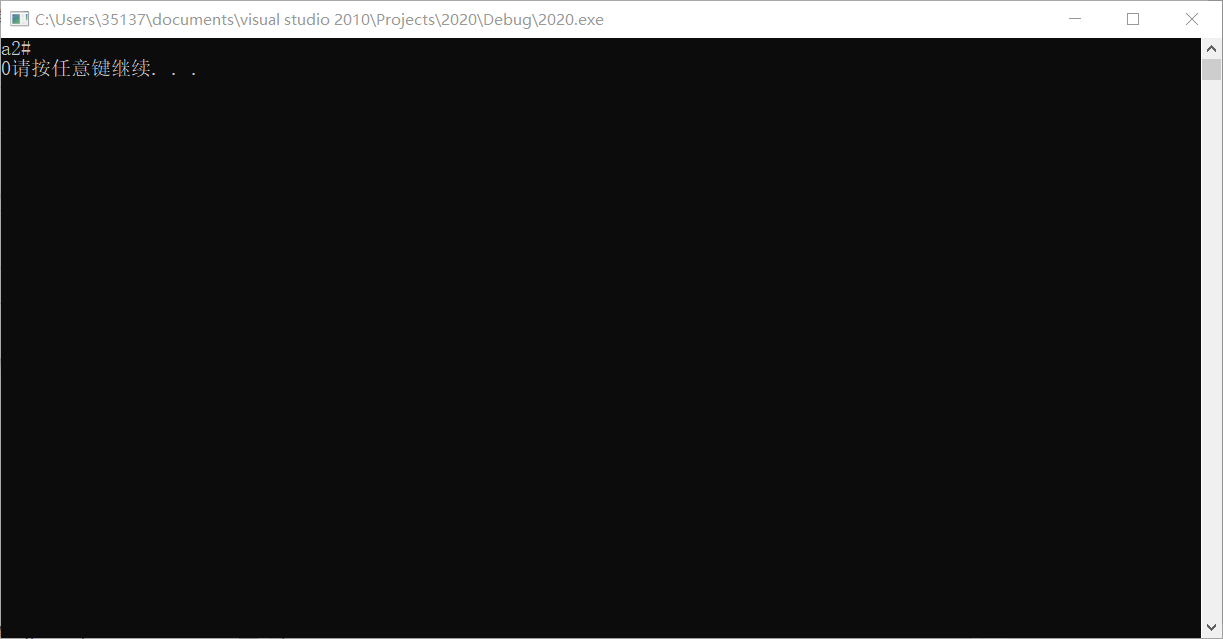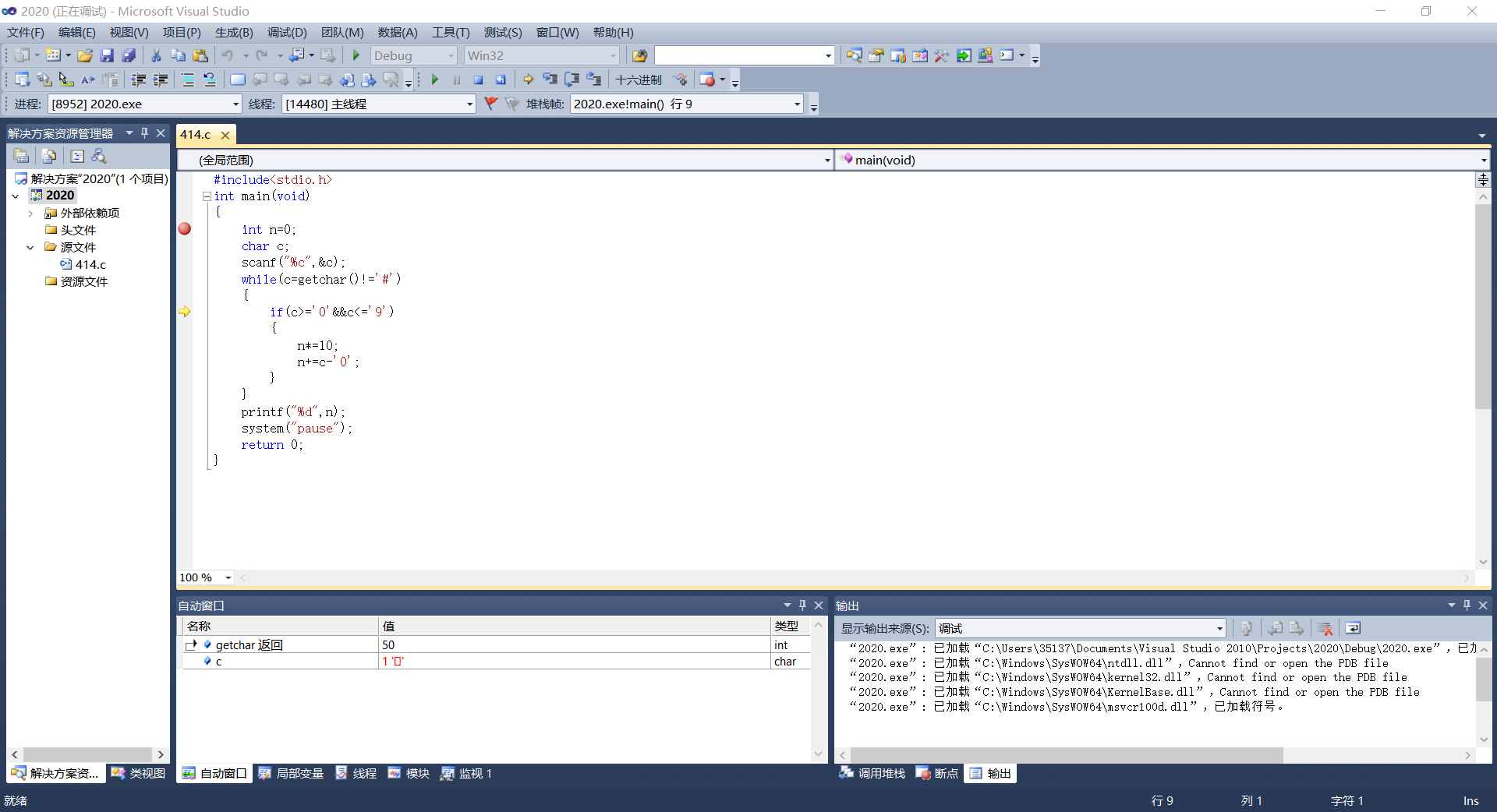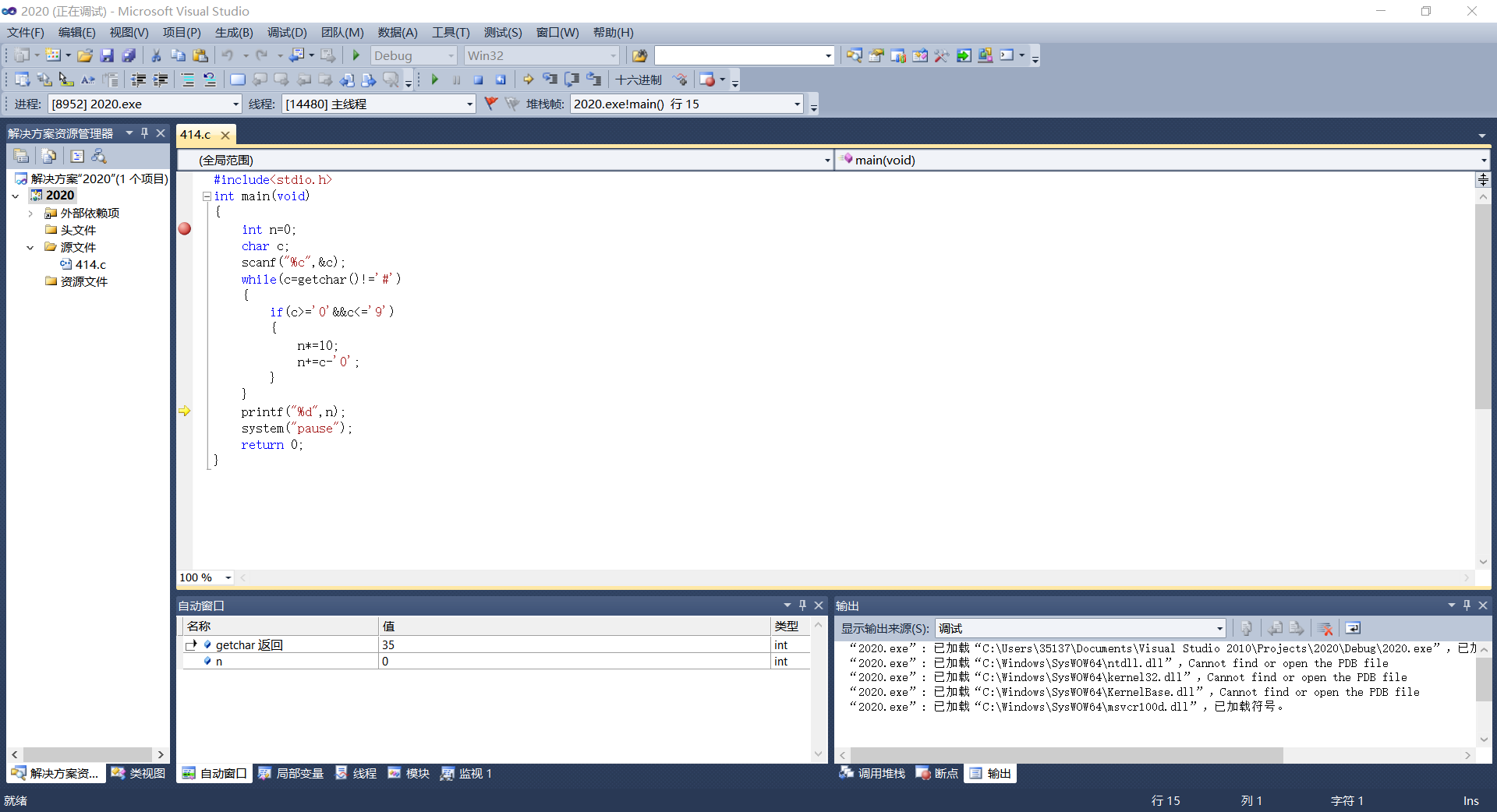``````int main(void)
{
int n=0;
char c;
scanf("%c",&c);
while((c=getchar()!)='#')
{
if(c>='0'&&c<='9')
{
n*=10;
n+=c-'0';
}
}
printf("%d",n);
system("pause");
return 0;
}

``````

``````(c=getchar()!='#')
``````

，代码如下

``````int main(void)
{
int n=0;
char c;
scanf("%c",&c);
while(c=getchar()!='#')
{
if(c>='0'&&c<='9')
{
n*=10;
n+=c-'0';
}
}
printf("%d",n);
system("pause");
return 0;
}

``````1个回答

(c=getchar()!='#')

((c=getchar())!='#')